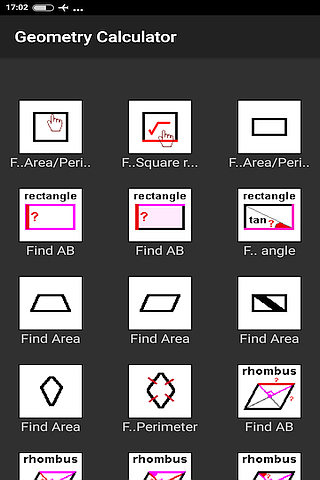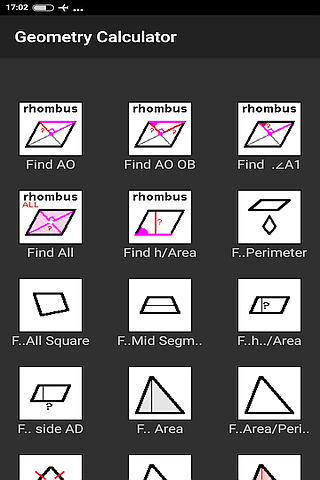ALL FREE.
More than 100 samples.
Squares and triangles area and perimeter.
Pythagorean theorem.
Circle Rhombuses and Kites.
volume of rectangular pyramid end cube formula.
Formula for the lateral surface area of a cylinder.
Radius of circle in a triangle.
Bisector of an angle of a triangle.
coordinate plane with points.
Bug Fix